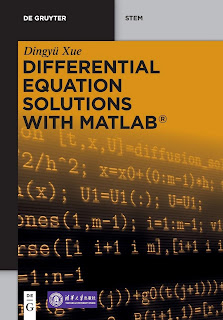APPLIED MATHEMATICS

# Differential Equation Solutions With MATLAB Fundamentals and Numerical Implementations PDF Free DownloadAnalytical solutions of differential equations are explored first, followed by the numerical solutions of different types of ordinary differential equations (ODEs), as well as the universal block diagram based schemes for ODEs. Boundary value ODEs, fractional-order ODEs and partial differential equations are also discussed.